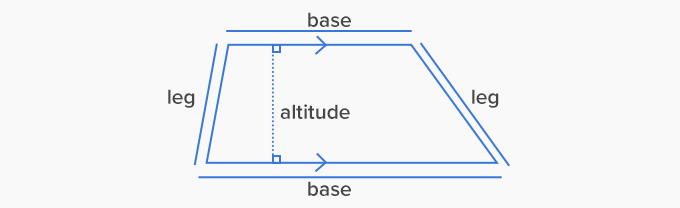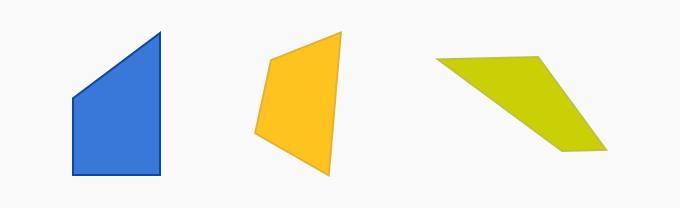# Trapezoid - Definition with Examples

The Complete K-5 Math Learning Program Built for Your Child

• 40 Million Kids

Loved by kids and parent worldwide

• 50,000 Schools

Trusted by teachers across schools

• Comprehensive Curriculum

Aligned to Common Core

What is Trapezoid?

A trapezoid, also known as a trapezium, is a flat closed shape having 4 straight sides, with one pair of parallel sides.

The parallel sides of a trapezium are known as the bases, and its non-parallel sides are called legs. A trapezium can also have parallel legs. The parallel sides can be horizontal, vertical or slanting.

The perpendicular distance between the parallel sides is called the altitude.Examples:Non-Examples:Types of trapezoid

Trapezoid is of three types namely

1. Right trapezoid: It has a pair of right angles.2. Isosceles trapezoid: It has equal length of non-parallel sides. In the image, sides, AD and BC are equal.3. Scalene trapezoid: It neither has equal angles nor has equal sides.Properties of a trapezoid

• A trapezoid is a parallelogram if both pairs of its opposite sides are parallel.

• A trapezoid is a square if both pairs of its opposite sides are parallel; all its sides are of equal length and at right angles to each other.

• A trapezoid can be a rectangle if both pairs of its opposite sides are parallel; its opposite sides are of equal length and are at right angles to each other.

Real life Examples

A few out of many trapezoid examples are the face of a popcorn box, handbag, and bridges.Fun Facts Trapezoid was known as τραπέζιον ‘trapézion’ in Ancient Greek which literally means “a little table” and also refers to “irregular quadrilateral”. Also, ‘oid’ in Ancient Greek means “resembling”. The word trapezium was introduced in the English language in1570. Marinus Proclus was the first person to use the word trapezoid in the first book of Euclid’s Elements.

Won Numerous Awards & Honors